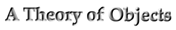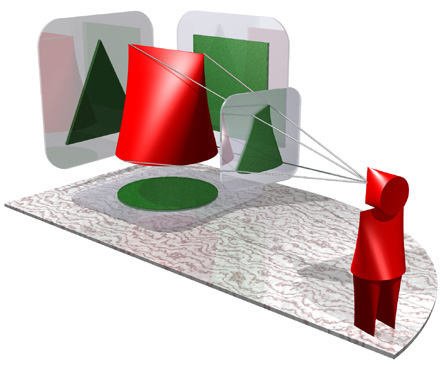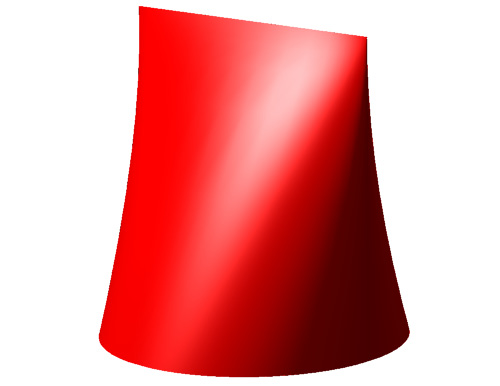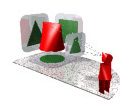﻿ A Theory of Objects - Object Subject

#### "Trisquirclehedron"

Let it be known far and wide that Jack C. Wileden has named this object in a fair contest.### "Object Disorientation": QuickTime Movie

#### Construction

Start with a circle on the horizontal plane. Place a square on a vertical plane with its base matching a diameter of the circle. Place a triangle on an orthogonal vertical plane with its base matching a diameter of the circle, and with its tip on the upper edge of the square. Each horizontal cross-section of the solid is given by an ellipse-like curve with its major axis given by the intersection of the cross-section plane with the square, and its minor axis given by the intersection of the cross-section plane with the triangle.

Questions: what are the interesting choices of ellipse-like curves? (E.g.: real ellipses. The drawing above uses splines.) What are the properties of the resulting solids? (E.g., surface, volume, convexity, vertical cross-sections, alternative characterizations.)